PSAT Math : Whole and Part

Example Questions

← Previous 1

Example Question #1 : Whole And Part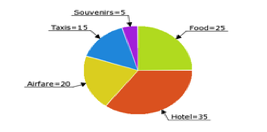David's trip expenses are pictured in the above pie chart (numbers = % of his total expenses). If he spent $75 on taxis, how much did he spend on hotel and souvenirs combined? Possible Answers:$175

$250$225

$200$40

$200 Explanation: David spent$75 on taxis, which were 15% of his total expenses on the trip. He therefore spent 75(100/15) = $500 on the trip altogether. The hotel and souvenirs make up 35% + 5% = 40% of his total expenses. 40% of 500 is$200.

Example Question #2 : Whole And Part

30% of what number is 20?

Solve to the nearest hundredth.

0.67

1.5

66.67

150%

66.67

Explanation:

This is a very basic form percentage question. This can be rewritten:

0.3 * x = 20

(Remember, the word "of" in a word problem indicates multiplication, while the word "is" indicates an equals sign).

Solve for x: x = 20 / 0.3 = 66.67

A toy is on sale for 43% off. Its sale price is $21.37. What is the full price? Possible Answers: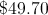None of the available answers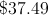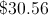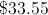Correct answer:Explanation: An algebraic expression for this item is: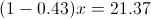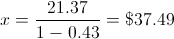Example Question #4 : Whole And Part Twenty-six students planned to contribute an equal amount to purchase a gift for their teacher. After 18 students had paid, they had collected$76.50. What is the total price of the gift?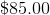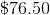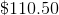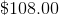Explanation:

If $76.50 had been collected after 18 students had paid, we can determine how much each student contributed:$76.50/18 = $4.25 per student Now we can multiply this by the total number of students (26) to get the full price of the gift: 26 x$4.25 = $110.50 Example Question #5 : Whole And Part If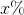ofis, then what isof? Possible Answers: 50 100 200 20 10 Correct answer: 50 Explanation: The first part of the problem tells us that x% of 20 is 50. We can model x% as x/100 or 0.01x. To find x% of 20, we can multiply 0.01x and 20. In other words, we can write the following equation: (0.01x)(20) = 50 Divide both sides by 20. 0.01x = 2.5 Divide both sides by 0.01. x = 250. The question then asks us to find 20% of x. We can represent 20% as 0.2, and we know that x is 250. Therefore, 20% of 250 = 0.2(250) = 50. The answer is 50. Example Question #6 : Whole And Part Ifof a number is, what isof the number? Possible Answers: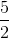Correct answer:Explanation: Let x be the number in question. Then 2/3 * x = 18. x = 18 * 3/2 = 27 Now find 1/9 of 27: 1/9 * 27 = 3 Example Question #7 : Whole And Part A salesperson for a car dealership earns 16% commission on all sales. She has sold one vehicle this week, a$82,000 SUV on Tuesday, and today is Saturday. She would like to earn a total of $10,000 in commissions by week's end. At the very least, how much in sales must she accomplish today to meet her goal? Possible Answers: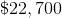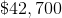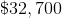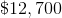She has already met her goal. Correct answer: She has already met her goal. Explanation: The salesperson has already earned a commission of 16% of$82,000, which is equal to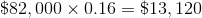.

This exceeds her goal for the week of \$10,000, so she has already reached her goal.

Example Question #1 : How To Find The Part From The Whole With Percentage

Your friend has 100 pounds of bacon and offers to share 45% of it with you.  If you promised your mom 30% and your cousin 25% of your share, how many pounds of bacon do you end up with?

45 pounds

13.5 pounds

24.75 pounds

11.25 pounds

20.25 pounds

20.25 pounds

Explanation:

Your share = 45% of 100 pounds of bacon = .45 * 100 = 45 pounds

For mom and cousin = 30% + 25% =  55%

Percent left for you = 100% - 55% = 45%

45% of 45 pounds = .45 * 45 = 20.25 pounds

Example Question #2 : How To Find The Part From The Whole With Percentage

A container has ten liters of a twenty percent sucrose solution by volume. How many liters of the solution should be removed from the container and then replaced by the same volume of a forty percent sucrose solution, so that the final solution is ten liters of a twenty-five percent sucrose solution?

2

4

1.25

0.5

2.5

2.5

Explanation:

The final solution is going to have a concentration of twenty-five percent and a volume of 10 liters. The amount of sucrose in the solution is equal to the concentration (expressed as a decimal) multiplied by the total volume. Therefore, the final solution will have 25% • 10 = 0.25 • 10 = 2.5 liters of sucrose.

Let x be the amount of the solution that is removed and then replaced by the forty percent solution. The amount of twenty percent solution that will remain will be equal to 10 – x, because the final solution will still have ten liters. (The amount removed is replaced with the same amount, so the final volume of the solution is the same.)

So we have to get 2.5 liters of sucrose from the twenty percent solution and the forty percent solution combined. The amount of sucrose we will get from the twenty percent solution equals the volume of the twenty percent solution (which we established will be 10 – x) multiplied by twenty percent as a decimal.

Amount of sucrose from 20% solution = 0.20 • (10 – x)

The amount of sucrose we get from the forty percent solution will equal the product of x and forty percent (since we defined x as the amount of forty percent solution).

Amount of sucrose from 40% solution = 0.40x

Now, we need to add the amount of sucrose from the 20% and the 40% solutions, and then set this equal to 2.5, because ultimately we will have 2.5 liters of sucrose in the solution. We can write the equation as follows and then solve for x:

0.20 • (10 – x) + 0.40x = 2.5

Here is a table that we can also use to summarize the information discussed above.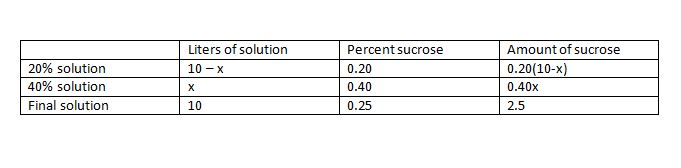0.20 • (10 – x) + 0.40x = 2.5

Distribute.

2 – 0.2x + 0.4x = 2.5

Combine x terms.

2 + 0.2x = 2.5

Subtract 2 from both sides.

0.2x = 0.5

Divide both sides by 0.2.

x = 2.5 liters

Example Question #3 : How To Find The Part From The Whole With Percentage

A total of 200,000 votes were cast for two opposing candidates. If the losing candidate received 40% of the vote, how many votes did the winning candidate receive?

80,000

50,000

100,000

120,000